Games

There are 366 different Starters of The Day, many to choose from. You will find below some starters on the topic of Games. A lesson starter does not have to be on the same topic as the main part of the lesson or the topic of the previous lesson. It is often very useful to revise or explore other concepts by using a starter based on a totally different area of Mathematics.

Main Page

Games Starters: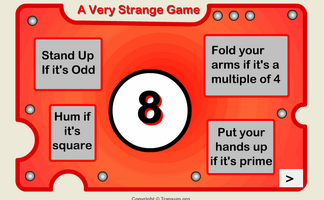Four different actions depending on the number which appears.A game for two players requiring a calculator and thinking skills.A game in which players take turns to add a single-digit number to what is already in the calculator. The winner is the player who makes the display show 30.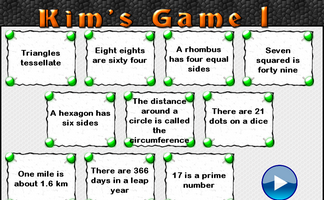This is the Maths version of the traditional memory game. Memorise 10 mathematical facts then recall them as they disappear from view.This is the Maths version of the traditional memory game. Memorise 10 mathematical facts then recall them as they disappear from view.This is the Maths version of the traditional memory game. Memorise 10 mathematical facts then recall them as they disappear from view.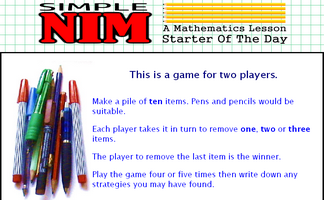The classic game of Nim played with a group of pens and pencils. The game can be extended to the multi-pile version.

Exercises23 or Bust

A game involving mental arithmetic and strategy for two players or one player against the computer.Algebra Pairs

The classic Pelmanism or pairs game requiring you to match equivalent expressions.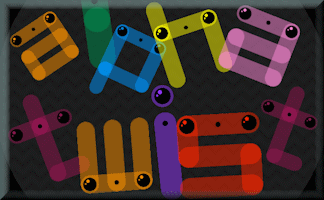Alpha Twist

Develop your skills and understanding of rotation in this fast-paced challenge.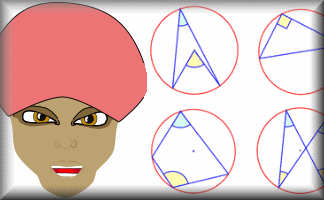Angle Theorem Kim's Game

A memory game to be projected to help the whole class revise the circle angle theorems.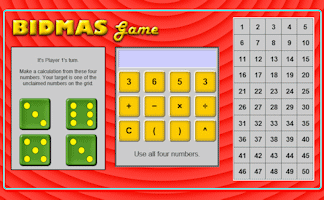BIDMAS Game

An online interactive game celebrating the order of mathematical operations.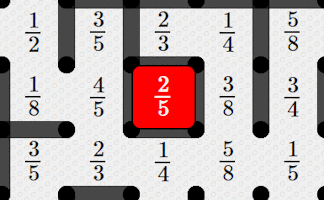Boxed In Fractions

The classic dots and boxes two-player game with the addition of some fractions which determine your score.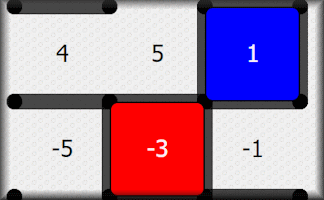Boxed In Numbers

The classic dots and boxes game with the addition of some positive and negative numbers which determine your score.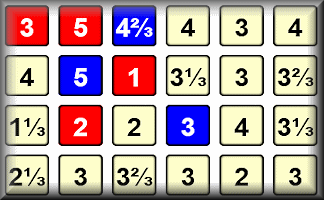This is a game for two players. You should know how to find the mean, median and range of a set of numbers.Circle Pairs

Find the matching pairs of circle diagrams and circle properties in this interactive online game.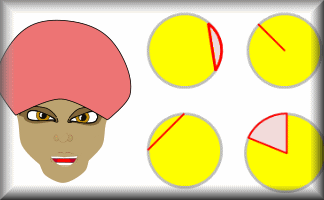Circle Parts Kim's Game

A memory game to be projected to help the whole class revise the names for the parts of a circle.Circle Theorem Pairs

A pairs game based around ten theorems about the angles made with chords, radii and tangents of circles.Clock Times Pairs

The traditional pairs or Pelmanism game adapted to test the ability to compare analogue and digital times.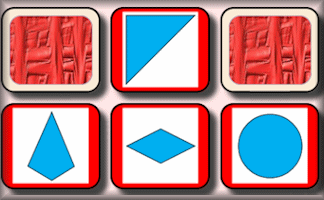Congruent Pairs

Match the congruent shapes, those with the same shape and size.Connect 4 Factors

This a game for one or two players. The winner is the first to line up four numbers with a common factor.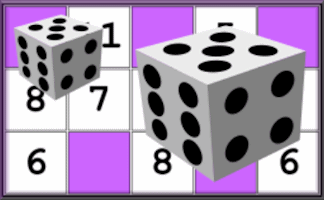Dice Bingo

Choose your own numbers for your bingo card. The caller uses two dice and adds the numbers together.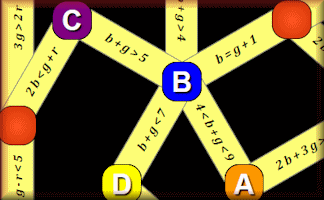DiceGebra

An online board game for two players evaluating algebraic equations and inequalities.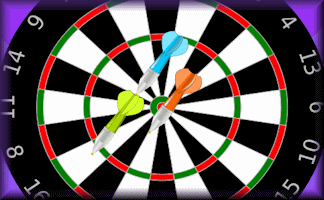Digital Darts

An online darts game for one or two players requiring skill, strategy and mental arithmetic.Discombobulated

A fun game requiring you to find numbers which add up to the target number as quickly as possible.Don't Shoot The Square

You will need to be quick on the draw to shoot all of the numbers except the square numbers.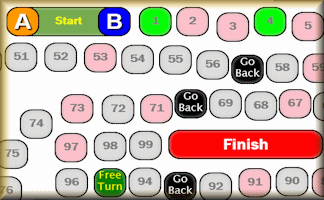Dump-A-Dice Race

An online board game for two players involving prime and square numbers and making choices.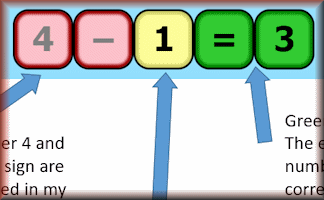Equatero

Find the expression from a series of guesses and clues.Equivalent Fraction Pairs

The traditional pairs or Pelmanism game adapted to test knowledge of equivalent fractions.Fifteen

A strategy game. Play against the computer to select three numbers that add up to 15.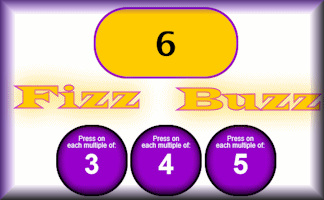Fizz Buzzer

The digital version of the popular fizz buzz game. Press the buzzers if they are factors of the counter.Flabbergasted Game

This game for one or two players is an exciting challenge to demonstrate an understanding of factors and multiples.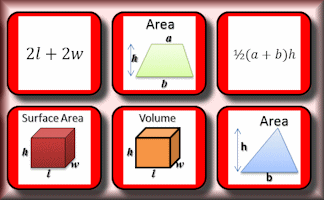Formulae Pairs

Find the matching pairs of diagrams and formulae for basic geometrical shapes.Fraction Decimal Pairs

The traditional pairs or Pelmanism game adapted to test knowledge of simple fractions and their equivalent decimals.Fraction Percentage Pairs

The traditional pairs or Pelmanism game adapted to test knowledge of simple fractions and their equivalent percentages.Fractions Kim's Game

A memory game to be projected to help the whole class recognise basic fractions.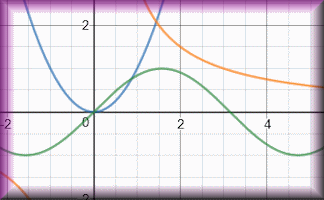Graph Equation Pairs

Match the equation with its graph. Includes quadratics, cubics, reciprocals, exponential and the sine function.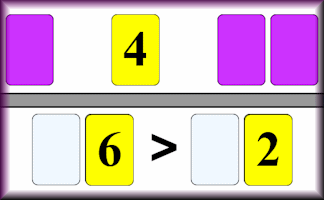Great Expectation

An interactive online activity requiring logical thinking and a certain amount of luck to place the digits on the correct side of the inequality sign.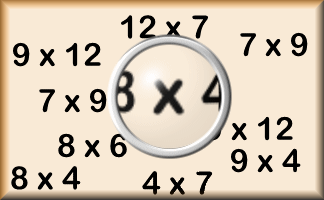Hard Times

The hardest multiplication facts (according to Transum research) are presented in the form of pairs games.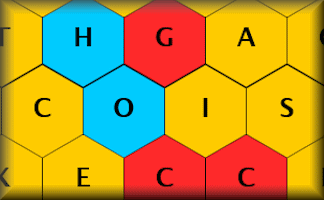Hexblock Hunter

The mathematical version of TV's Blockbusters game show. Find the mathematical words from the clues.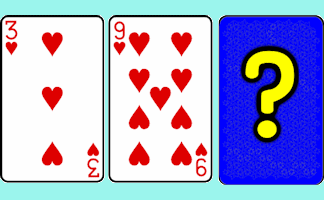Hi-Low Predictions

A version of the Play Your Cards Right TV show. Calculate the probabilities of cards being higher or lower.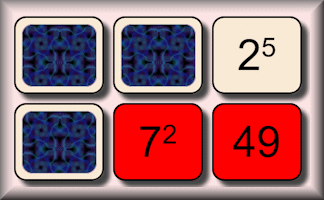Indices Pairs

The traditional pairs or pelmanism game adapted to test knowledge of indices.Loci Construction Pairs

Match the description of the loci to the diagram of the construction.Manifest

An exciting and thought-provoking number placing game for two players or one player versus the computer.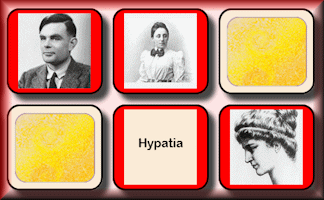Mathematician Pairs

The traditional pairs or Pelmanism game requiring the ability to recognise some of the great mathematicians.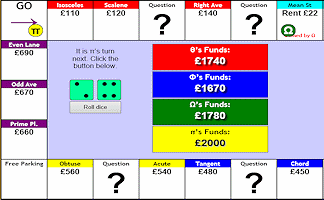Mathopoly

A game of buying and selling property with maths questions thrown in for good measure.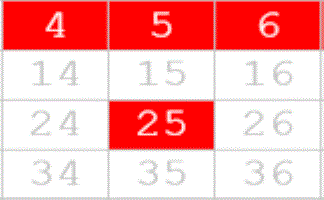Maths Bingo

This application will pick the bingo numbers for you and present each of the numbers as a mathematical expression.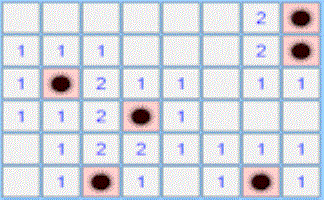Mine Find

Find where the mines are hidden without stepping on one.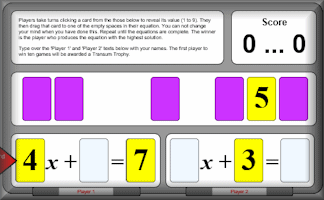Nevertheless

Players decide where to place the cards to make an equation with the largest possible solution.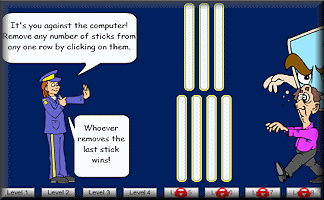Nim

Nim is a mathematical game of strategy in which two players take turns removing objects from groups of objects.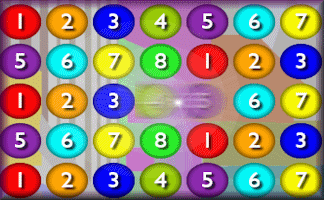Number Crunch Saga

A lively numeracy game requiring you to align three numbers to create the given target sum or product.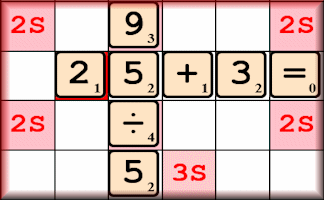Numerate

Earn points by creating equations on the grid in this game of equations.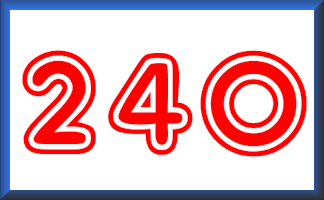Pairs 240

Find the pairs of numbers that multiply together to give a product of 240 in this collection of matching games.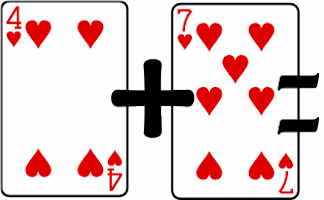Pairs Eleven

Find pairs of playing cards of the same suit which add up to eleven.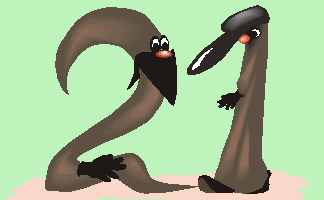Pairs Twenty One

Find the pairs of numbers that add up to 21 in this collection of matching games.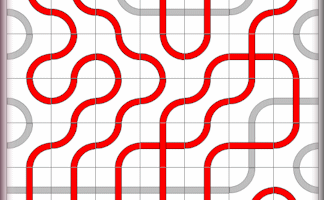Path Puzzle

A great puzzle requiring you to use all of the cards to create a continuous red line from start to finish.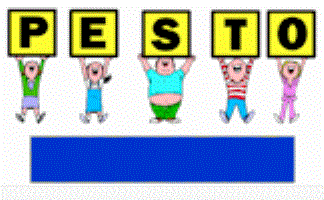Pesto

Students classify numbers randomly appearing on the screen by holding up cards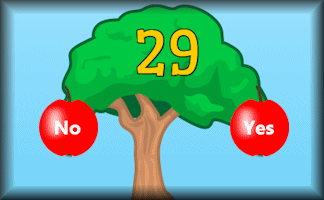Pick The Primes

Pick the prime fruit from the tree as quickly as possible. Practise to improve your personal best time.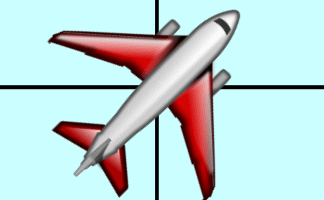Plane Bearings

A visual aid designed to help pupils estimate three figure bearings.Playing Card Maths

Imagine you are on a desert island with nothing but a pack of playing cards. Do you have to stop learning mathematics?Polybragging

The Transum version of the Top Trumps game played online with the properties of polygons.Prime Pairs Game

A game for two players who take turns to select two numbers that add up to a prime number.Prime Pips in Pots

This is a version of Wari, one of the oldest known games to still be widely played today. It involves small prime numbers.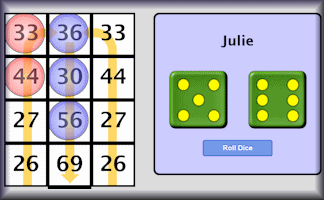Remainder Race

A game involving chance and choice requiring an ability to calculate the remainder when a two digit number is divided by a single digit number.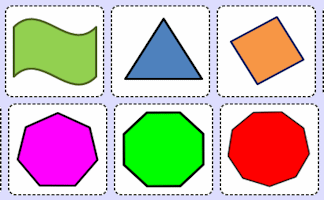Rotational Symmetry Pairs

The traditional pairs or pelmanism game adapted to test knowledge of rotational symmetry.Rounding Snap

If the last card put down equals the previous card to the nearest whole number then all players race to shout SNAP!Shark's Dinner

The mathematical version of the classic hangman game. Guess the letters that are contained in the words.Skunk

A game for the whole Maths class to play involving chance and choice.Snail Race

A race between 12 snails. Which snail is most likely to win? This is the students' version of the race simulation.Snooker Angles

An online game for one or two players requiring an ability to estimate angles.Songkran Game

Where should you stand in the circle to win the Songkran game?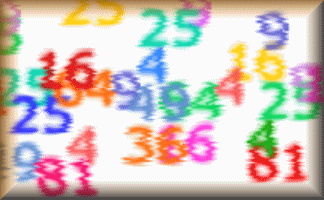Square Pairs Game

A game for two players who take turns to select two numbers that add up to a square number.Squorder

The Transum version of the traditional sliding tile puzzle.Sum Game

A game against the clock to find the numbers which add up to the target number.Superior

A game for two players who compete to make the largest possible number from randomly selected cards.Tables Conga

Use the arrow keys to collect all the multiples in order while avoiding the Conga Virus!Tables Dash

Revise multiplication facts by racing across the screen to match the times tables question with the correct answer without getting hit by lightning.Tables Grab

A one or two player game. The objective is to grab all the multiples of the chosen times table faster than the other player.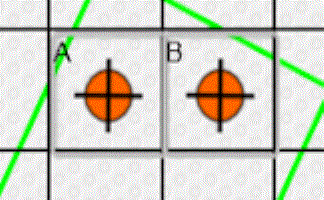Tantrum

A game, a puzzle and a challenge involving counters being placed at the corners of a square on a grid.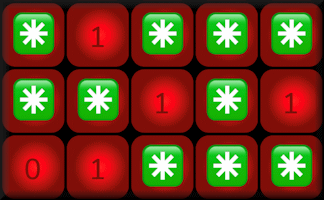The Rowcol Game

A strategy game based on claiming tokens in specific rows and columns.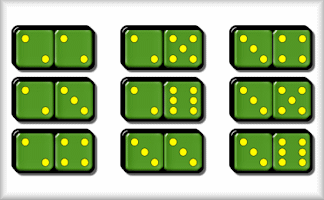Threes and Fives

A game for two players who take turns to add a domino to the line in order to score threes and fives.Tran Towers

An adventure game requiring students to solve puzzles as they move through the old mansion.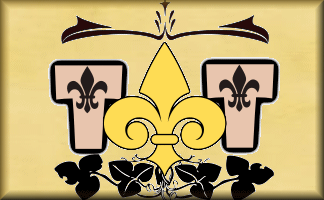Tran Tunnels

A mini adventure game containing maths puzzles and problems. Find your way through the maze of tunnels to find Goldberg's magic harpsicord.Transformation Tetris

Develop your skills translating and rotating shapes in this fast paced classic game.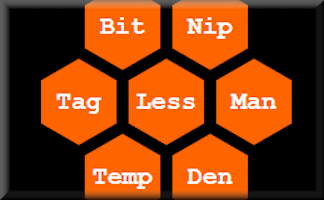Trilett

A strategy game requiring you to select three words with a common letter before the computer does.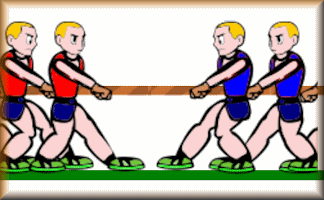Tug of War

A game for two players or teams testing their speedy reactions to mental arithmetic questions.Venn Diagram Pairs

The traditional pairs or pelmanism game adapted to test knowledge of Venn diagrams.Vocabero

Find the mathematical word from a series of guesses and clues.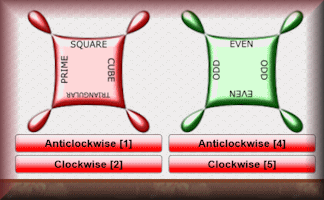Rotate the cogs to catch the flying numbers in the correct sections.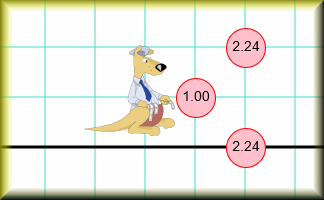Where's Wallaby?

Find the hidden wallaby using the clues revealed at the chosen coordinates.Yes No Questions

A game to determine the mathematical item by asking questions that can only be answered yes or no.

Search

The activity you are looking for may have been classified in a different way from the way you were expecting. You can search the whole of Transum Maths by using the box below.

Have today's Starter of the Day as your default homepage. Copy the URL below then select
Tools > Internet Options (Internet Explorer) then paste the URL into the homepage field.

Set as your homepage (if you are using Internet Explorer)

Do you have any comments? It is always useful to receive feedback and helps make this free resource even more useful for those learning Mathematics anywhere in the world. Click here to enter your comments.For All: